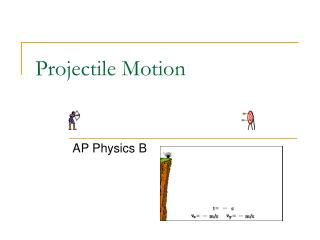Download PresentationProjectile MotionProjectile Motion - PowerPoint PPT Presentation

Download PresentationProjectile Motion
An Image/Link below is provided (as is) to download presentation

Download Policy: Content on the Website is provided to you AS IS for your information and personal use and may not be sold / licensed / shared on other websites without getting consent from its author. While downloading, if for some reason you are not able to download a presentation, the publisher may have deleted the file from their server.

- - - - - - - - - - - - - - - - - - - - - - - - - - - E N D - - - - - - - - - - - - - - - - - - - - - - - - - - -
Presentation Transcript

1. Projectile Motion AP Physics B

2. What is projectile? Projectile -Any object which projected by some means and continues to move due to its own inertia (mass).

3. Projectiles move in TWO dimensions Since a projectile moves in 2-dimensions, it therefore has 2 components just like a resultant vector. • Horizontal and Vertical

4. Horizontal “Velocity” Component • NEVER changes, covers equal displacements in equal time periods. This means the initial horizontal velocity equals the final horizontal velocity In other words, the horizontal velocity is CONSTANT. BUT WHY? Gravity DOES NOT work horizontally to increase or decrease the velocity.

5. Vertical “Velocity” Component • Changes (due to gravity), does NOT cover equal displacements in equal time periods. Both the MAGNITUDE and DIRECTION change. As the projectile moves up the MAGNITUDE DECREASES and its direction is UPWARD. As it moves down the MAGNITUDE INCREASES and the direction is DOWNWARD.

6. Combining the Components Together, these components produce what is called a trajectory or path. This path is parabolicin nature.

7. Horizontally Launched Projectiles Projectiles which have NO upward trajectory and NO initial VERTICAL velocity.

8. Horizontally Launched Projectiles To analyze a projectile in 2 dimensions we need 2 equations. One for the “x” direction and one for the “y” direction. And for this we use kinematic #2. Remember, the velocity is CONSTANT horizontally, so that means the acceleration is ZERO! Remember that since the projectile is launched horizontally, the INITIAL VERTICAL VELOCITY is equal to ZERO.

9. Horizontally Launched Projectiles Example: A plane traveling with a horizontal velocity of 100 m/s is 500 m above the ground. At some point the pilot decides to drop some supplies to designated target below. (a) How long is the drop in the air? (b) How far away from point where it was launched will it land? 1010 m 10.1 seconds

10. Vertically Launched Projectiles NO Vertical Velocity at the top of the trajectory. Vertical Velocity decreases on the way upward Vertical Velocity increases on the way down, Horizontal Velocity is constant

11. Vertically Launched Projectiles Since the projectile was launched at a angle, the velocity MUST be broken into components!!! voy vo q vox

12. Vertically Launched Projectiles There are several things you must consider when doing these types of projectiles besides using components. If it begins and ends at ground level, the “y” displacement is ZERO: y = 0

13. Vertically Launched Projectiles You will still use kinematic #2, but YOU MUST use COMPONENTS in the equation. voy vo q vox

14. Example A place kicker kicks a football with a velocity of 20.0 m/s and at an angle of 53 degrees. (a) How long is the ball in the air? (b) How far away does it land? (c) How high does it travel? vo=20.0 m/s q = 53

15. Example A place kicker kicks a football with a velocity of 20.0 m/s and at an angle of 53 degrees. (a) How long is the ball in the air? 3.26 s

16. Example A place kicker kicks a football with a velocity of 20.0 m/s and at an angle of 53 degrees. (b) How far away does it land? 39.24 m

17. Example A place kicker kicks a football with a velocity of 20.0 m/s and at an angle of 53 degrees. (c) How high does it travel? CUT YOUR TIME IN HALF! 13.01 m